/home /blog 31 Mar 2020 | Get ipynb

# Heat Exchange Network Training

A while back I found a gem of an article describing Neural Heat Exchanger. This post discusses an implementation of that technique.

We don't actually need backpropagation for this so Numpy will suffice for our work here. The basic idea is that of a heat exchanger. Imagine there are two pipes as so:

``````
┃  ┃          A           ┃  ┃
┃  ┃                      ┃  ┃
┃  ┗━━━━━━━━━━━━━━━━━━━━━━┛  ┃
┗━━━━━━━━━━━━━━━━━━━━━━━━━━━━┛
┏━━━━━━━━━━━━━━━━━━━━━━━━━━━━┓
┃  ┏━━━━━━━━━━━━━━━━━━━━━━┓  ┃
┃  ┃                      ┃  ┃
┃  ┃          B           ┃  ┃
``````

Hot water flows in pipe A from left to right and cold water flows in pipe B from right to left. If these pipes are touching, heat gets exchanged through contact.

The idea was to build two identical neural networks (feed forward), call them A and B. Now inputs are propagated through network A left to right and outputs are propagated through network B right to left. The weights in the network are updated locally and without a global update rule.

Let's first write code to make a simple feed forward network.

## Defining the network

We'll define a single Section class which allows us to lay down the "Pipe" in sections. It corresponds to a layer in the classic Feed Forward Network paradigm.

```# %pylab inline
plt.style.use("ggplot")
from tqdm import trange

def sigmoid(x):
return 1 / (1 + np.exp(-x))

class Section:
"""
Information always flows from network_input to right.
---->----->----

Build the pipe with sections like:

Section(3) < Section(3) < Section(3)
Section(3) > Section(3) > Section(3)

The < and > denote information flow and are used to build the sections.
"""

def __init__(self, dim):
self.dim = dim
self.network_input = None
self.right = None
self.ident = "[" + "".join(map(str, random.choice(range(10), 3))) + "]"

def takes_input(self, input):
self.network_input = input
self.weight = np.random.uniform(-1, 1, (input.dim, self.dim))

def __call__(self, input=None):
act = None
if self.network_input is not None:
act = self.network_input(input) @ self.weight
elif input is not None:
if not hasattr(
self, "weight"
):  # In case we haven't created the weights yet
self.weight = np.random.uniform(-1, 1, (input.shape, self.dim))
act = input @ self.weight

act = sigmoid(act)

self.act = act
return act

def __repr__(self):
return self.ident
```
```Populating the interactive namespace from numpy and matplotlib
```

This class defines a section of a Pipe. This is analogus to a layer in a neural network. With this definition we can define the pipes F for Forward and B for Backward like so.

```F = [Section(2) for _ in range(10)]
B = [Section(2) for _ in range(10)]
```

After creating the sections of the pipes we link the sections together using < and > operations. It's a nice way of defining relations between pipes. Any time you need to reorganize the pipe, you can just run a new "chain" of <>.

```for i in range(len(F)):
if i > 0:
F[i].takes_input(F[i - 1])
if i < (len(F) - 1):
B[i].takes_input(B[i + 1])
```

To propagate information through a pipe, we take the section where we want to collect output and call it with the input data which needs to go in at the other end of the pipe. For the forward pipe, we provide it with an instance of X and generate an instance of Y.

Let's try and predict if the numbers are within a circle of radius 0.8.

```x = np.random.uniform(-1, 1, (500, 2))
y = np.sqrt(np.power(x, 2).sum(axis=1)) <= 0.8
```

To visualize our data we can plot it to see the 1s and 0s.

```plt.plot(x[y, 0], x[y, 1], ".", label="One")
plt.plot(x[~y, 0], x[~y, 1], ".", label="Zero")
plt.legend()
```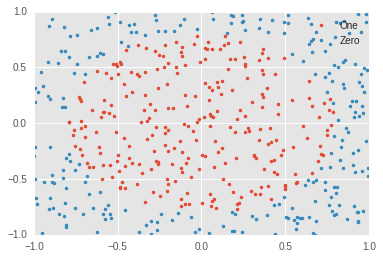```y = y.astype(float).reshape((-1, 1))
y = np.concatenate([y, y], axis=1).astype(float)

# For easiness sake we will simply duplicate the y signal to shape 2,2

out_section = F[-1]
predicted_output = out_section(x)
predicted_output
```
`array([0.57820847, 0.60292775])`

Similarly for the backward pipe, however here we provide it with an instance of Y and generate an instance of X

```out_section = B
predicted_input = out_section(y)
predicted_input
```
`array([0.61178636, 0.57108236])`

## Heat Exchange!

Now that we have the network defined and the "pipes" laid out, we can begin training this heat exchange network.

The update rule defined by Jürgen Schmidhuber says that each pipe section should try to minimize the distance between itself, it's previous section and to the corresponding section in the other pipe.

We'll first write a function which takes in a section and it's corresponding "input activation" and "side activation" and adds an update to it's weights. This is kind of like calculating gradients. For now we will keep in line with that method of doing things since we're already failiar with the loop of training which is as

1. Forward Pass
3. Weight Update

For now we will simply follow this loop.

```def update(net, prev_act, side_act, step):
"""
Update the network's weights to look more like the ones previous to it
and beside it
"""
update_prev = prev_act - net.act
update_side = side_act - net.act
total_update = update_prev + update_side

credit_assignment = net.weight / net.weight.sum(axis=0)
try:
total_update = total_update.reshape(credit_assignment.shape)
except:
pass

net.wt_update = credit_assignment * total_update * step
return net

def learn(F, B, a, b, step=0.001):
"""
This function updates the network on examples a and b
"""
# Calculate updates for F and B
F[-1](a)
B(b)
for i in range(len(F)):
if i == 0:
lastf = a  # Input was the last activation
else:
lastf = F[i - 1].act
if i == (len(F) - 1):
lastb = b  # output was the last activation
else:
lastb = B[i + 1].act
update(F[i], lastf, B[i].act, step)
update(B[i], lastb, F[i].act, step)
for f in F:
f.weight += f.wt_update
f.wt_update = None
for b in B:
b.weight += b.wt_update
b.wt_update = None
```
```weight_log = []
for epoch in trange(200):
weight_log.append([[n.weight.copy() for n in D] for D in [F, B]])
order = list(range(len(x)))
random.shuffle(order)
for i in order:
a, b = x[i], y[i]
learn(F, B, a, b)
```
```100%|██████████| 200/200 [00:45<00:00,  4.39it/s]
```

Let's see the weights of the network. What we'll do is plot the weights of the networks in a matrix and color by magnitude. For example in the matrix below, The top 2 rows belong to the weights of the Forward network and the bottom two rows belong to the backward network.

We can visualize them now.

```plt.style.use("ggplot")

def compare(a, b, only_after=True, cmap="Greens"):
i = a
rep = np.concatenate(
[np.concatenate(weight_log[i]), np.concatenate(weight_log[i])], axis=1
)
before = rep.T.copy()

i = b
rep = np.concatenate(
[np.concatenate(weight_log[i]), np.concatenate(weight_log[i])], axis=1
)
after = rep.T.copy()
if not only_after:
ax = plt.subplot(2, 1, 1)

plt.subplots(figsize=(15, 7))

ax.matshow(np.concatenate([before, after]), cmap=cmap)

plt.xlabel("Layer Weights")
plt.ylabel("[FB] at t=0 (lower),  [FB] at t=-1 (upper)")
plt.title("Lower4 = {}, Upper4 = {}".format(a, b))
ax = plt.subplot(2, 1, 2)
ax.matshow(after, cmap=cmap)
else:
plt.matshow(after, cmap=cmap)
plt.grid("off")
plt.title(str(b))

compare(0, 0)
```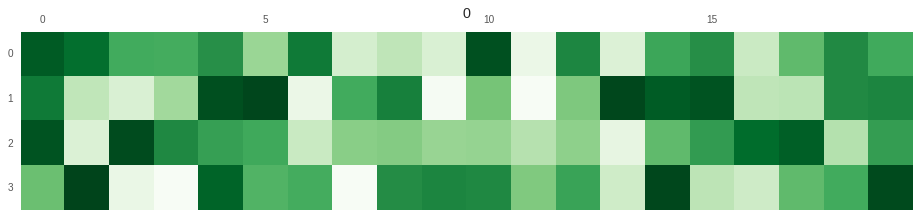Those were the random weights at the beginning. We'll see some more steps.

```compare(0, 1)
```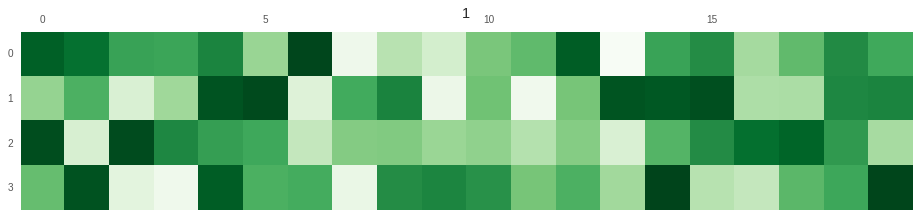```compare(0, 5)
```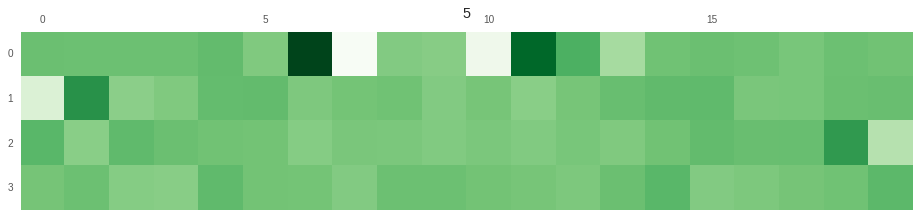```compare(0, -1)
```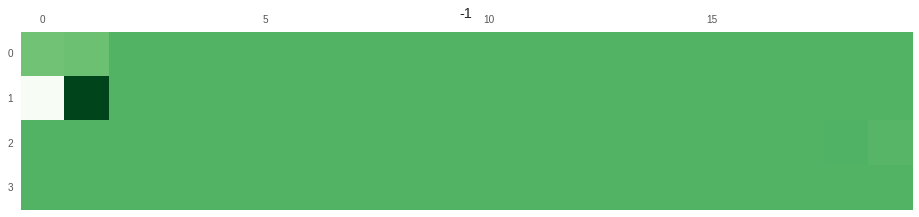We see some changes in the weights as the network learns.Perhaps a better way of seeing the change would be a video of the learning process. We will quickly save these images and create a video from them.

```!mkdir plots
for i in trange(len(weight_log)):
compare(0, i)
plt.savefig('plots/{}.png'.format(i))
plt.close()```
```100%|██████████| 200/200 [00:32<00:00,  6.17it/s]
```
```# %%capture
! rm test.mp4
! ffmpeg -r 10 -f image2 -s 700x200 -i plots/%d.png -vcodec libx264 -crf 25  -pix_fmt yuv420p test.mp4
! rm -rf plots```
`! mv test.mp4 ../videos/test.mp4`